# RD Sharma Solutions for Class 11 Chapter 28 - Introduction to Three Dimensional Coordinate Geometry Exercise 28.2

Download free pdf of RD Sharma Class 11 Maths Chapter 28 – Introduction to Three Dimensional Coordinate Geometry. Experts at BYJU’S have designed these solutions in a very clear manner that helps students solve problems in the most efficient possible ways. Securing good marks has become much easier by the usage of RD Sharma Class 11 Maths Solutions. In Exercise 28.2 we shall discuss problems based on the distance formula. Students can refer and download RD Sharma Class 11 Maths Solutions from the links provided below.

## Download the pdf of RD Sharma Solutions for Class 11 Maths Exercise 28.2 Chapter 28 – Introduction to Three Dimensional Coordinate Geometry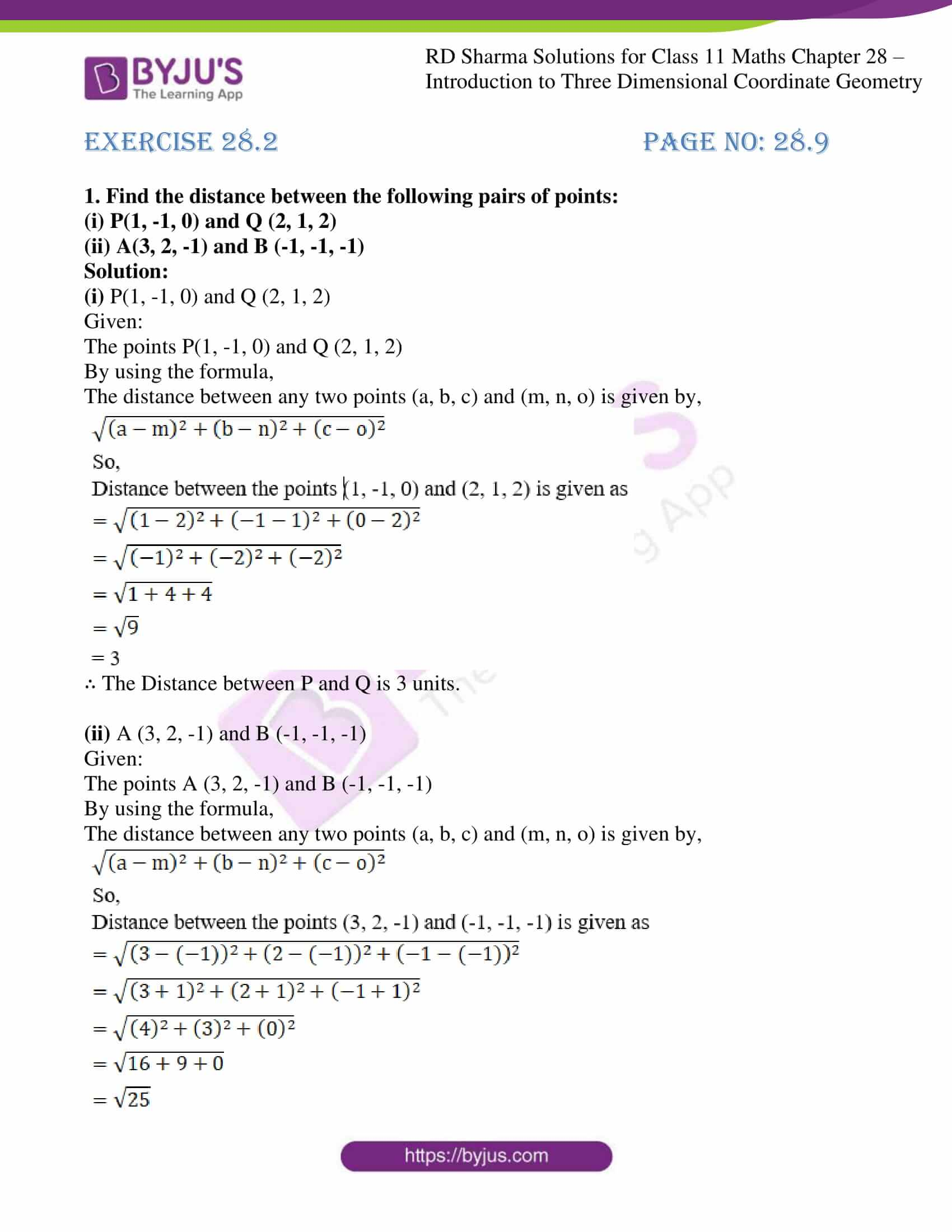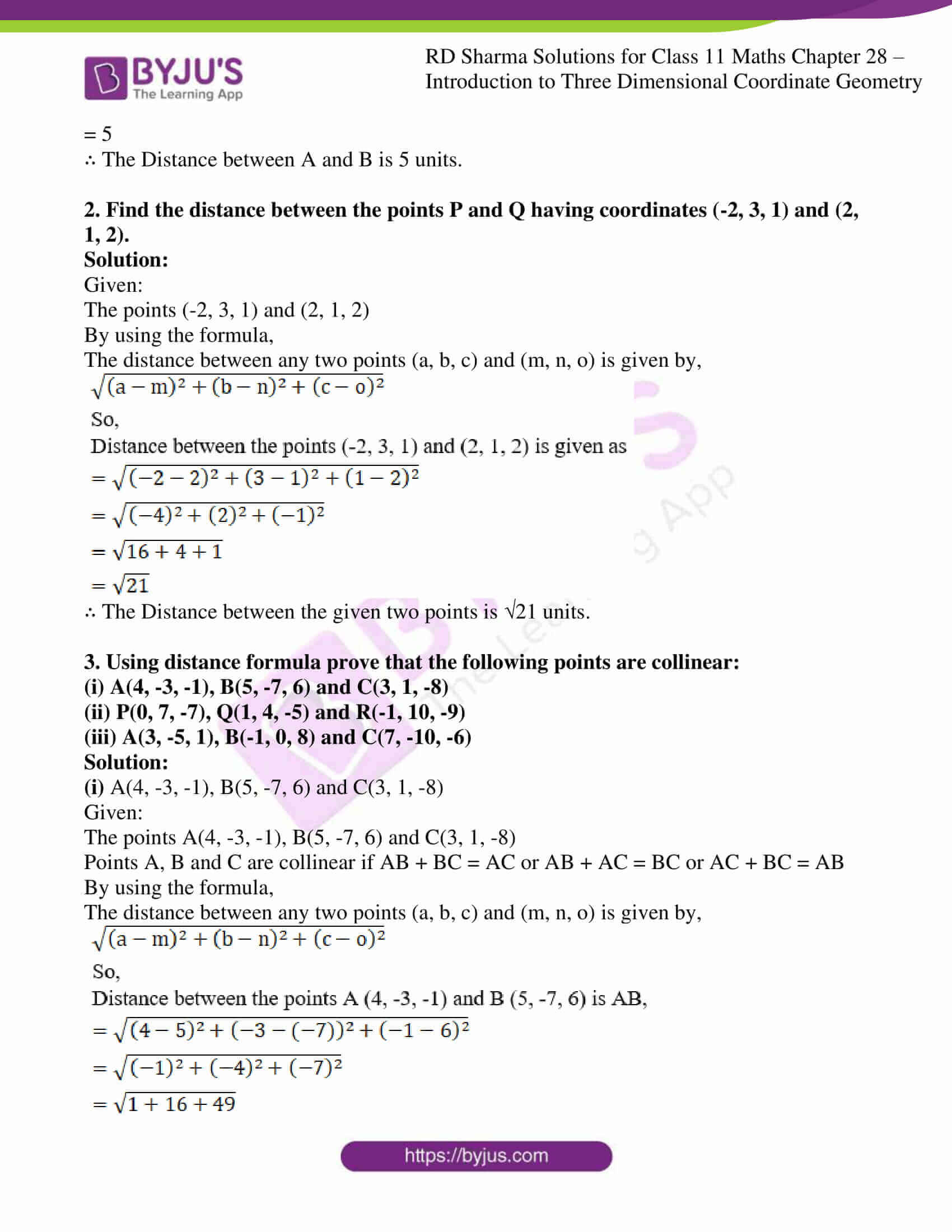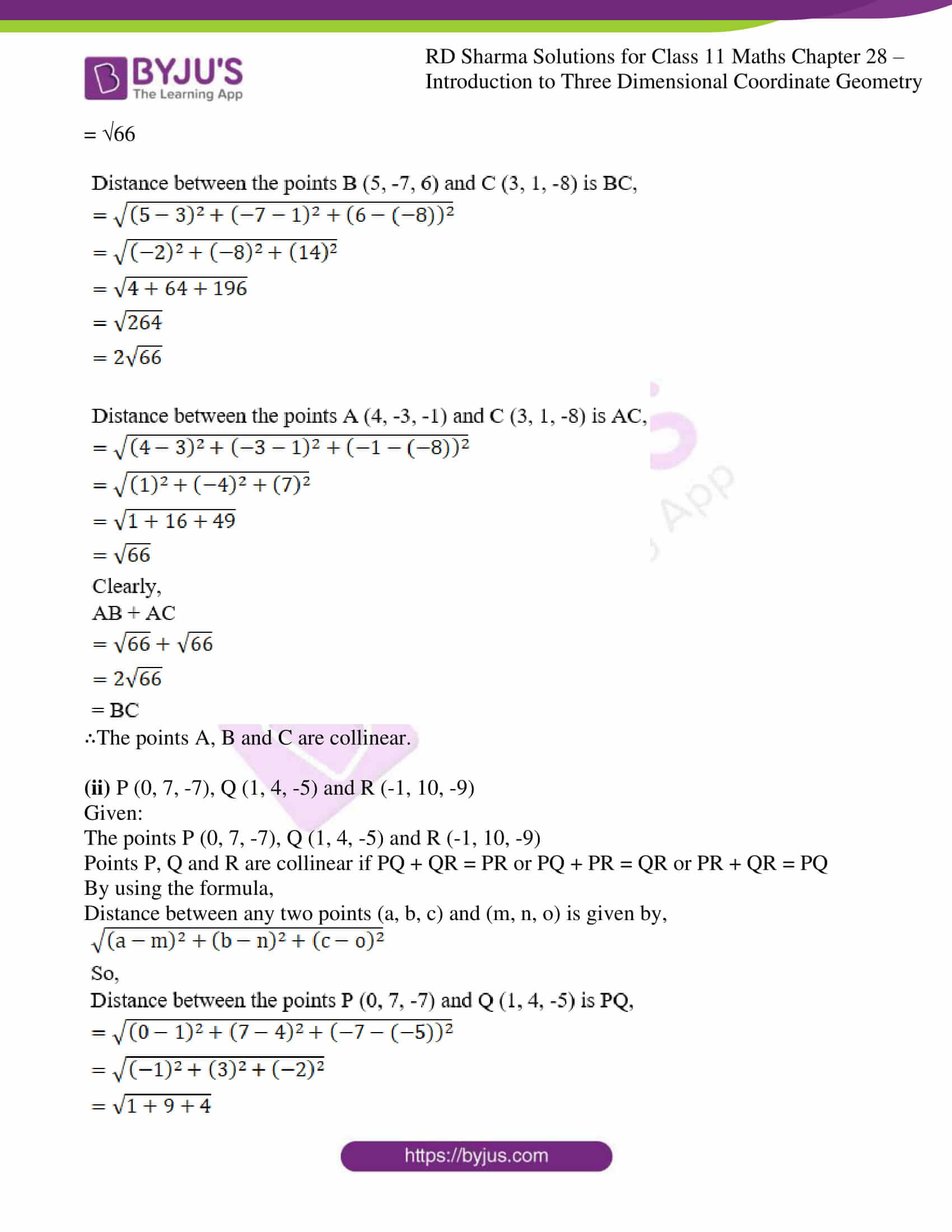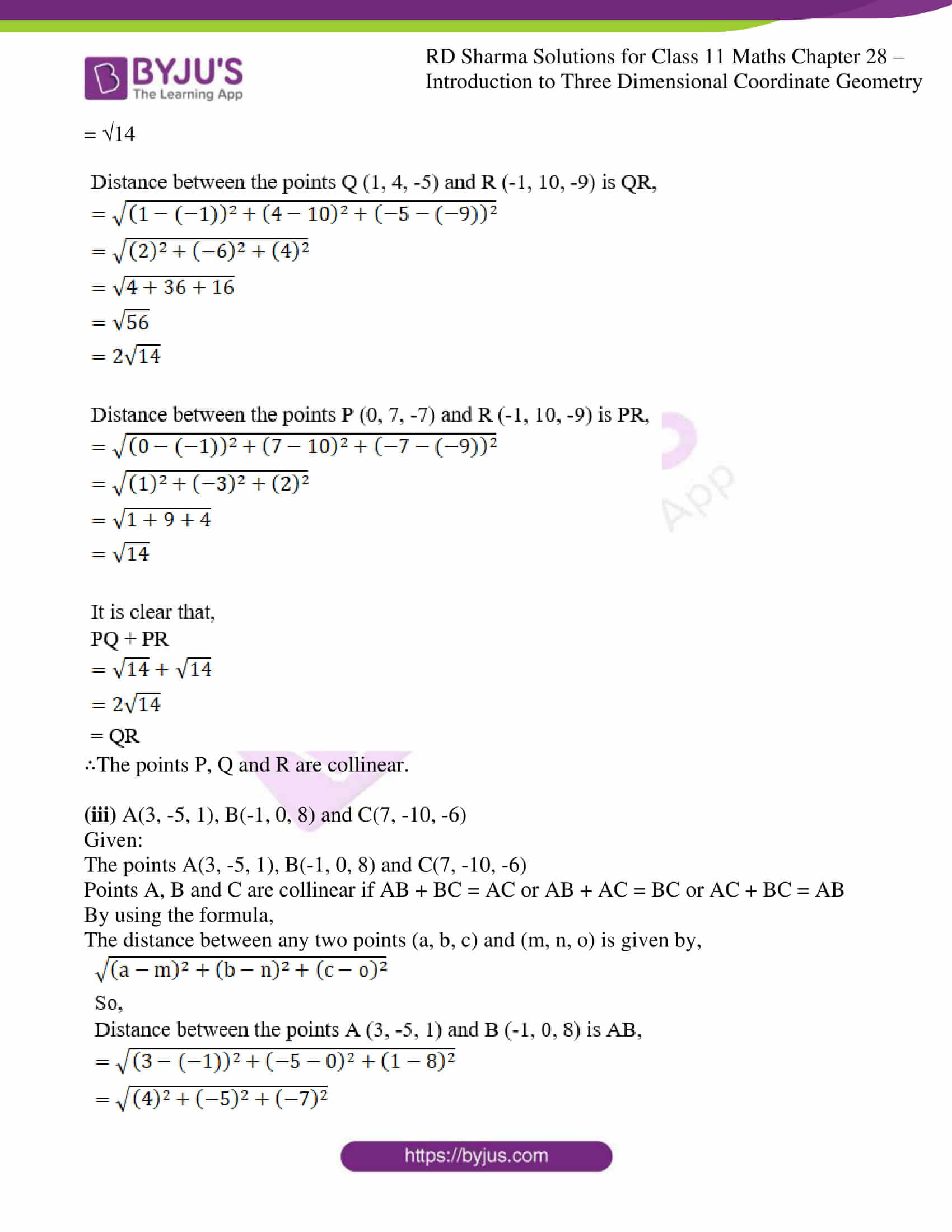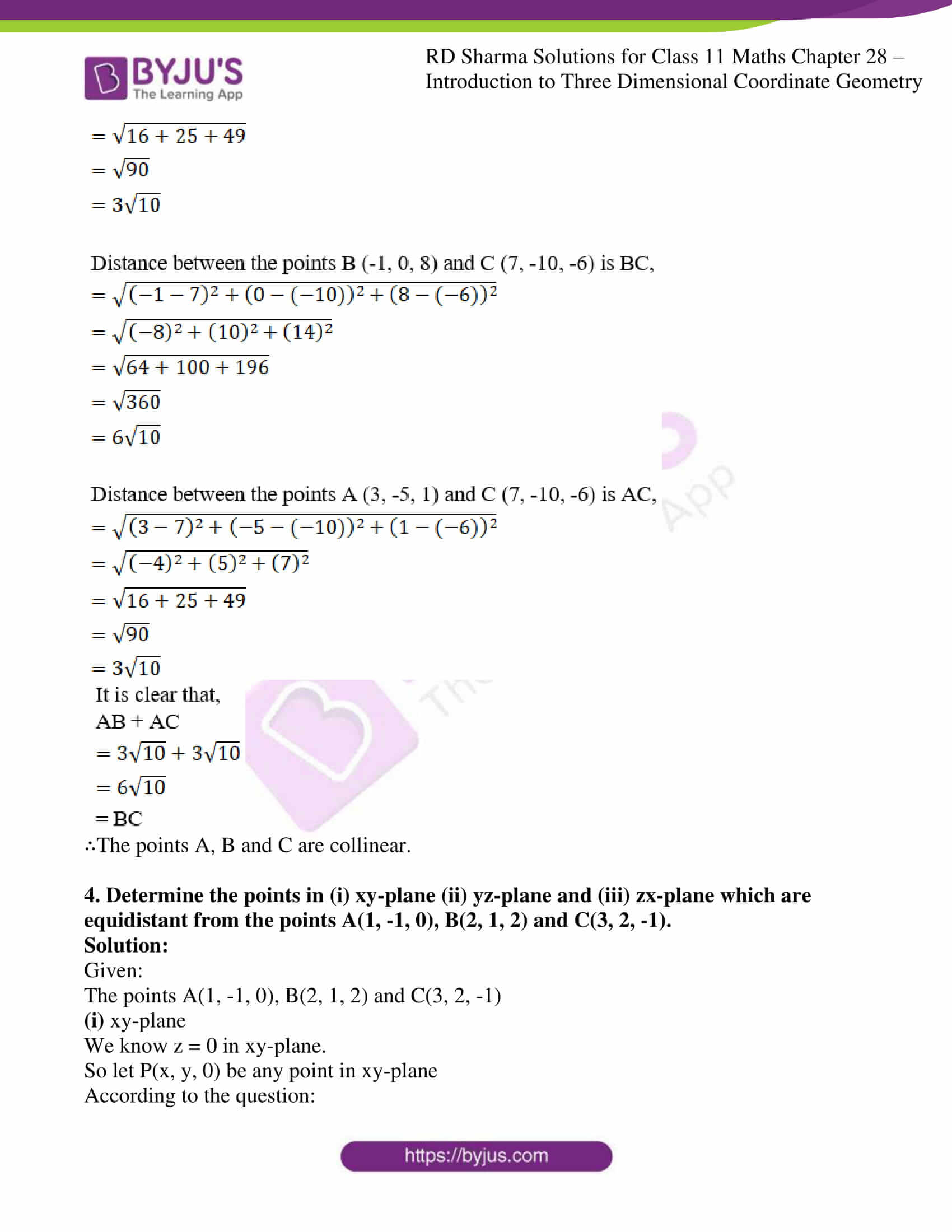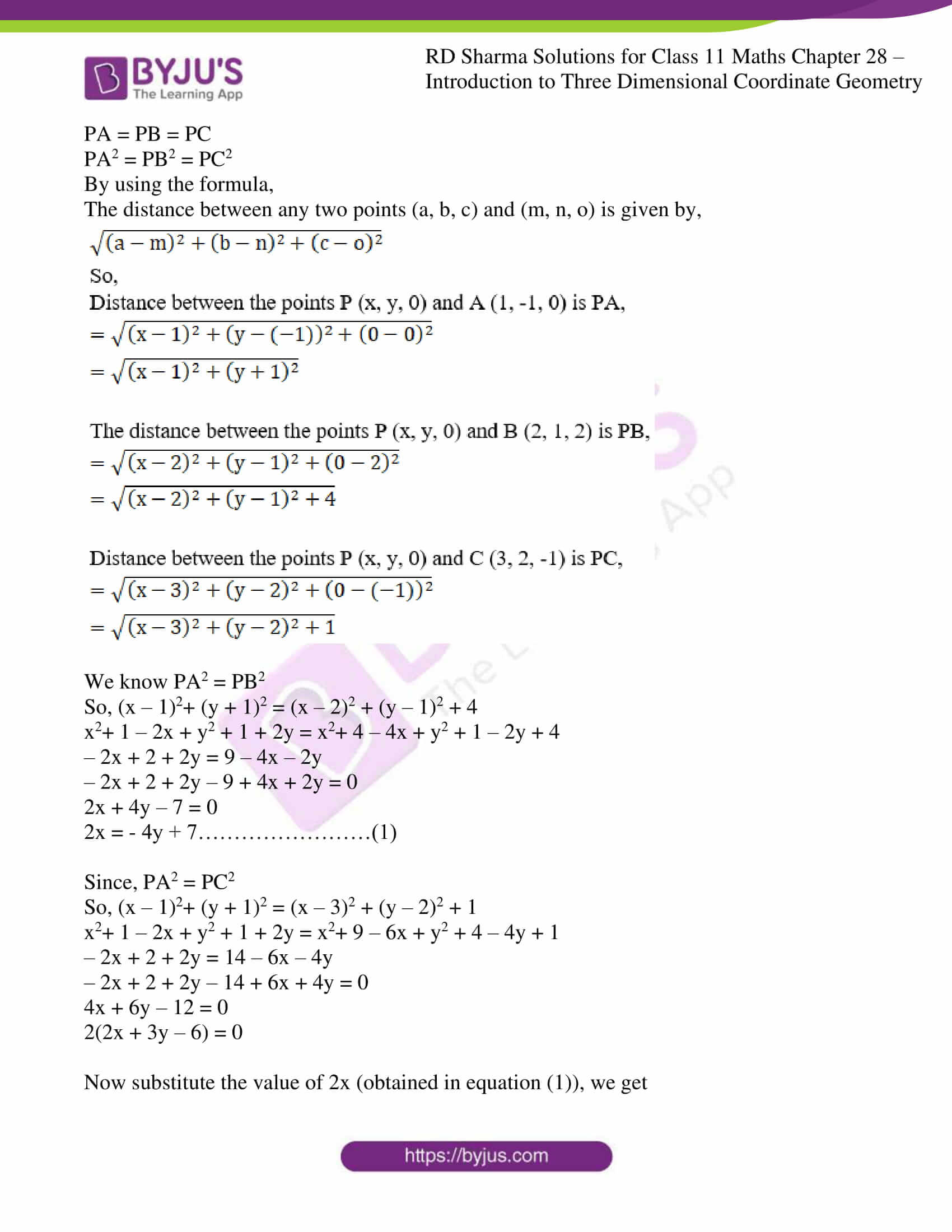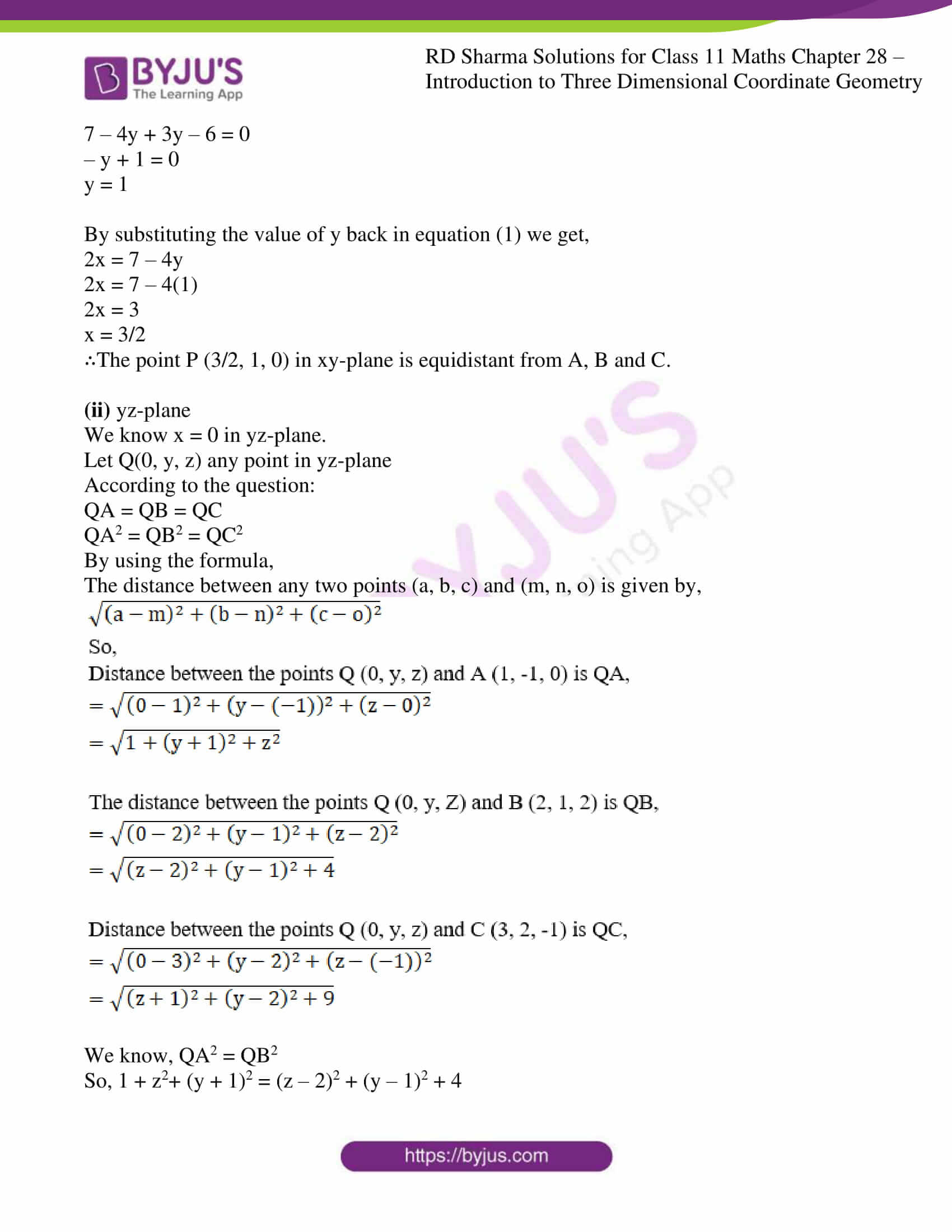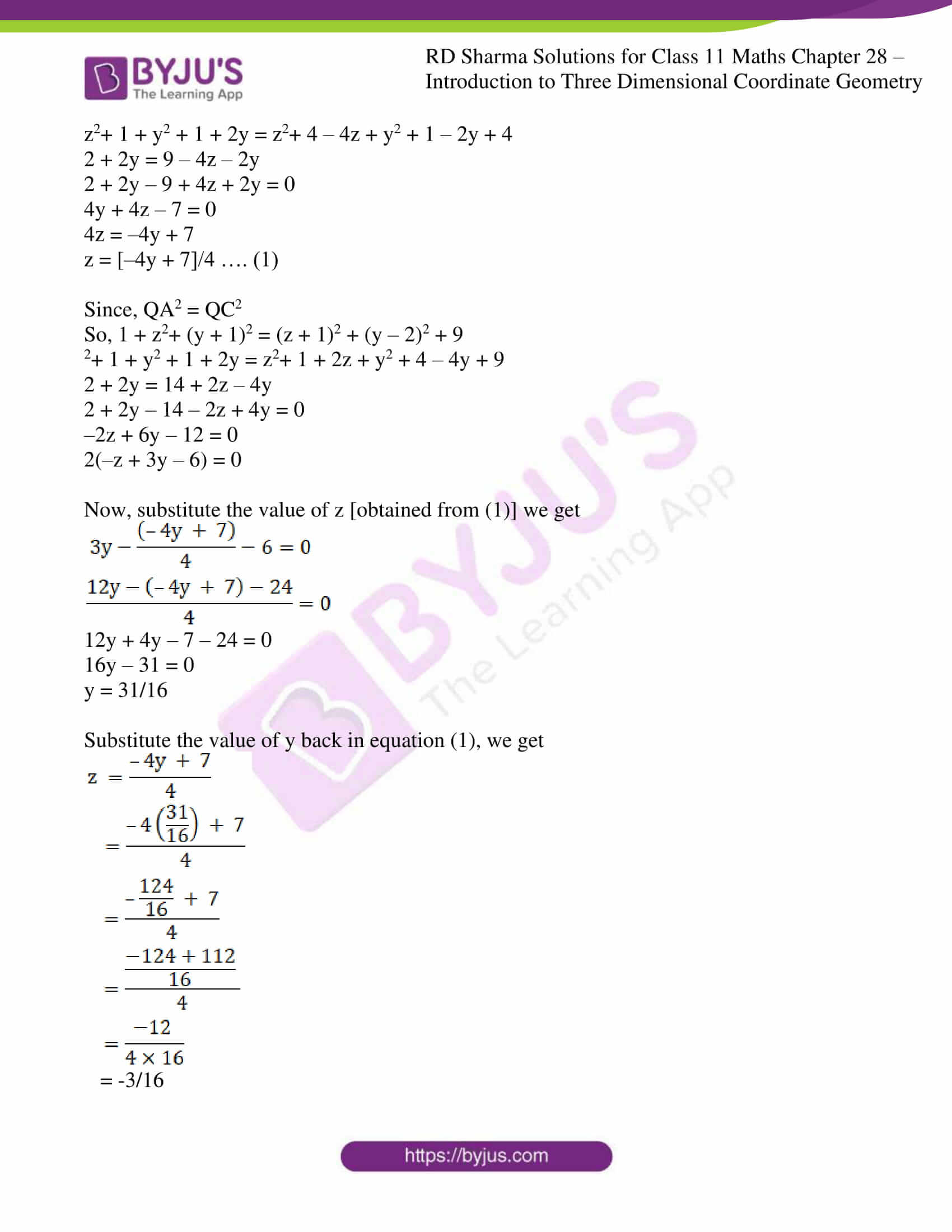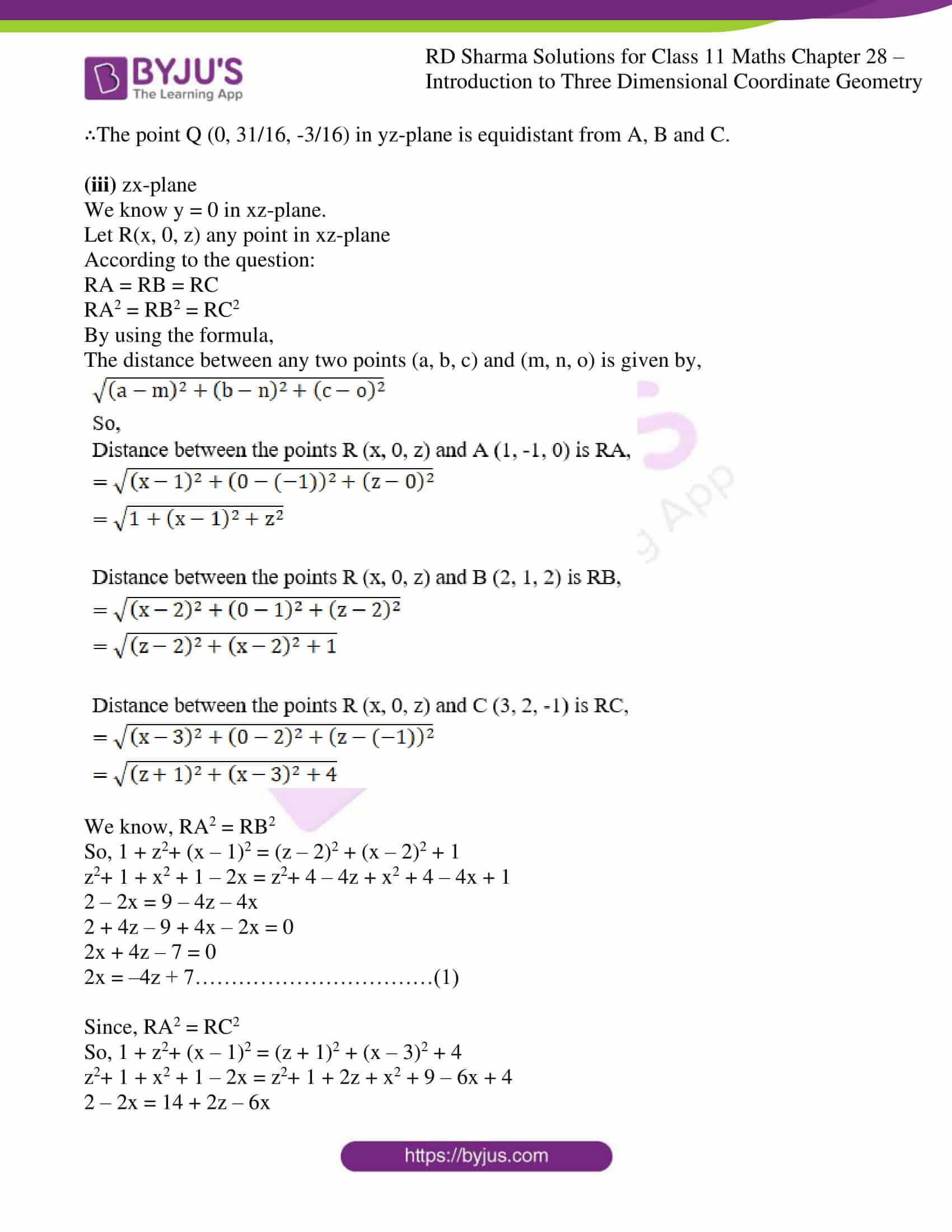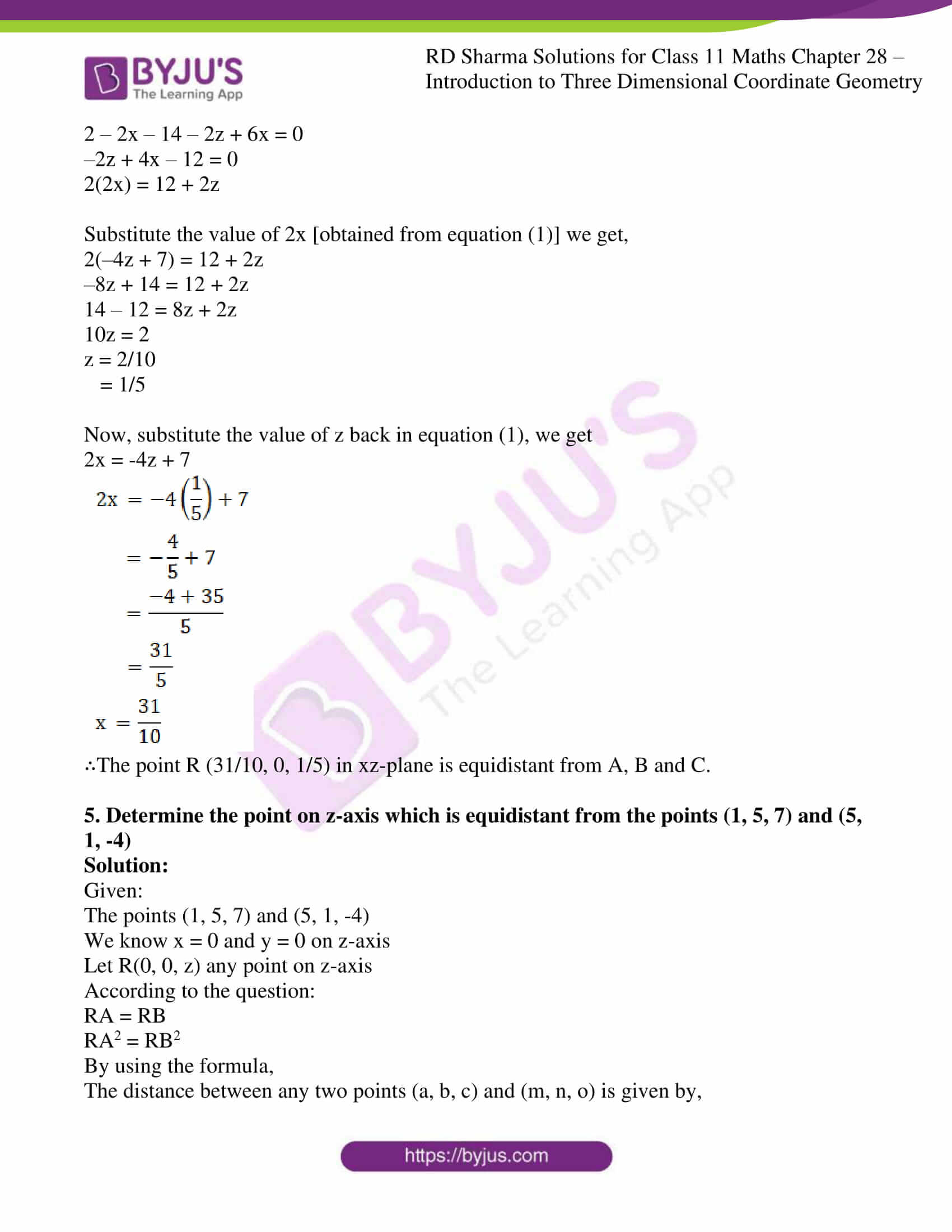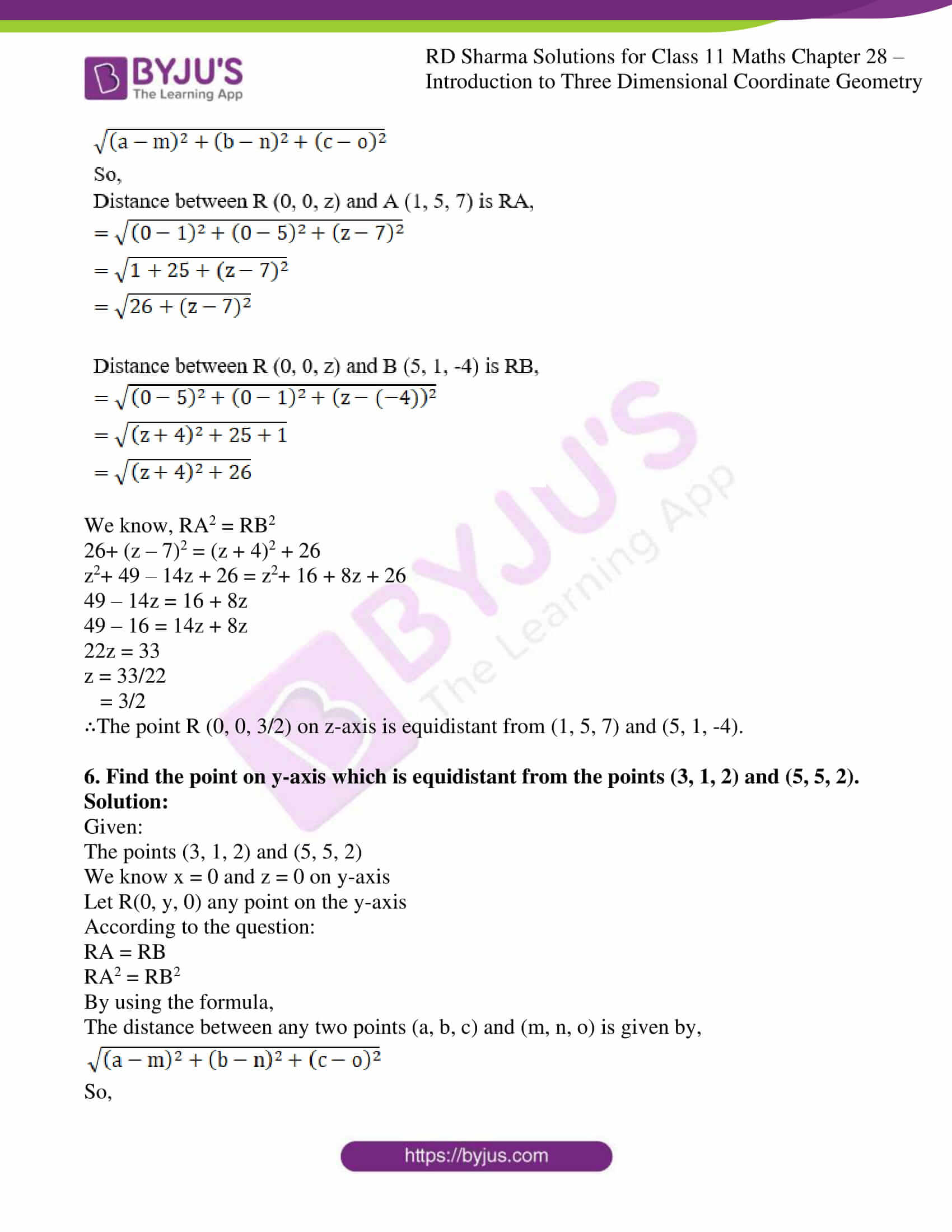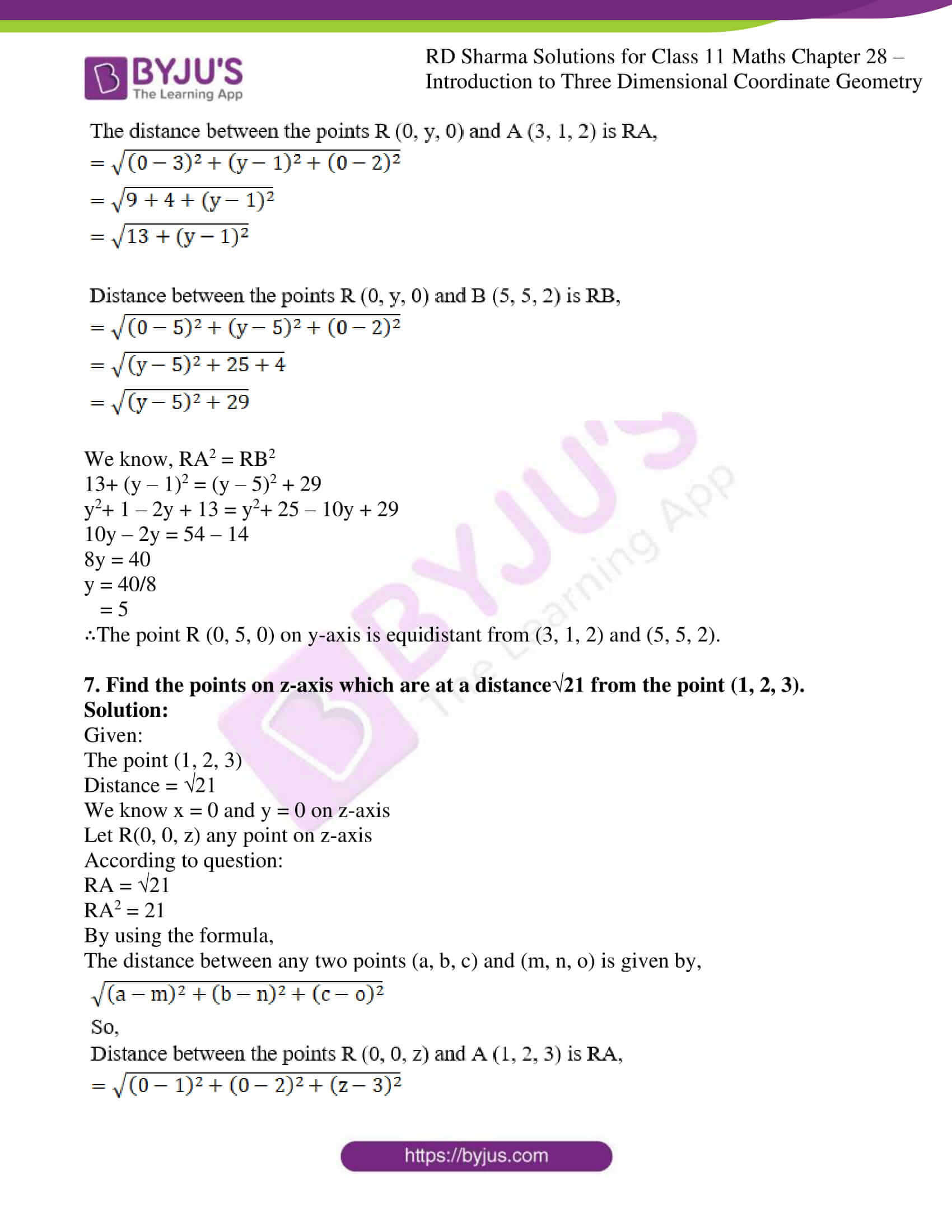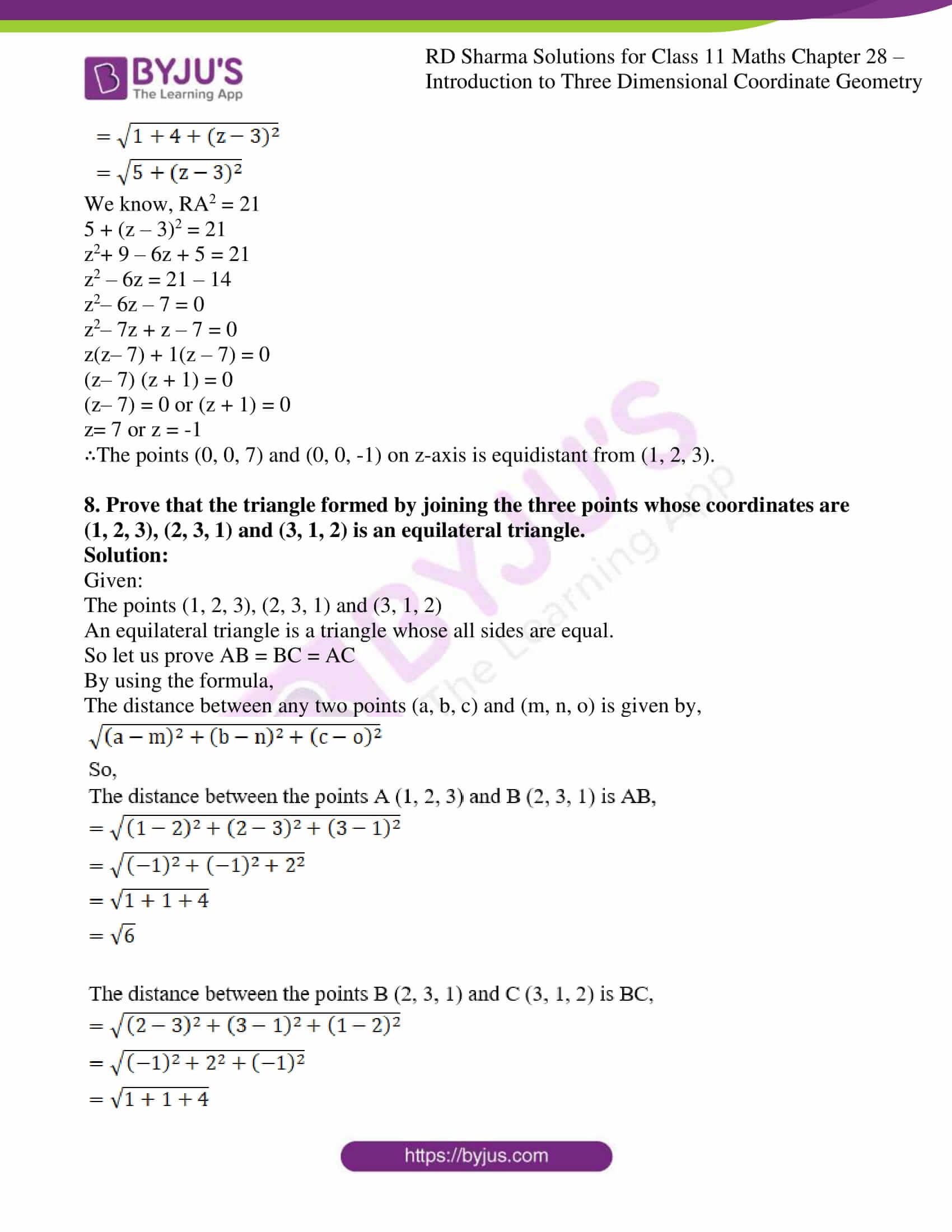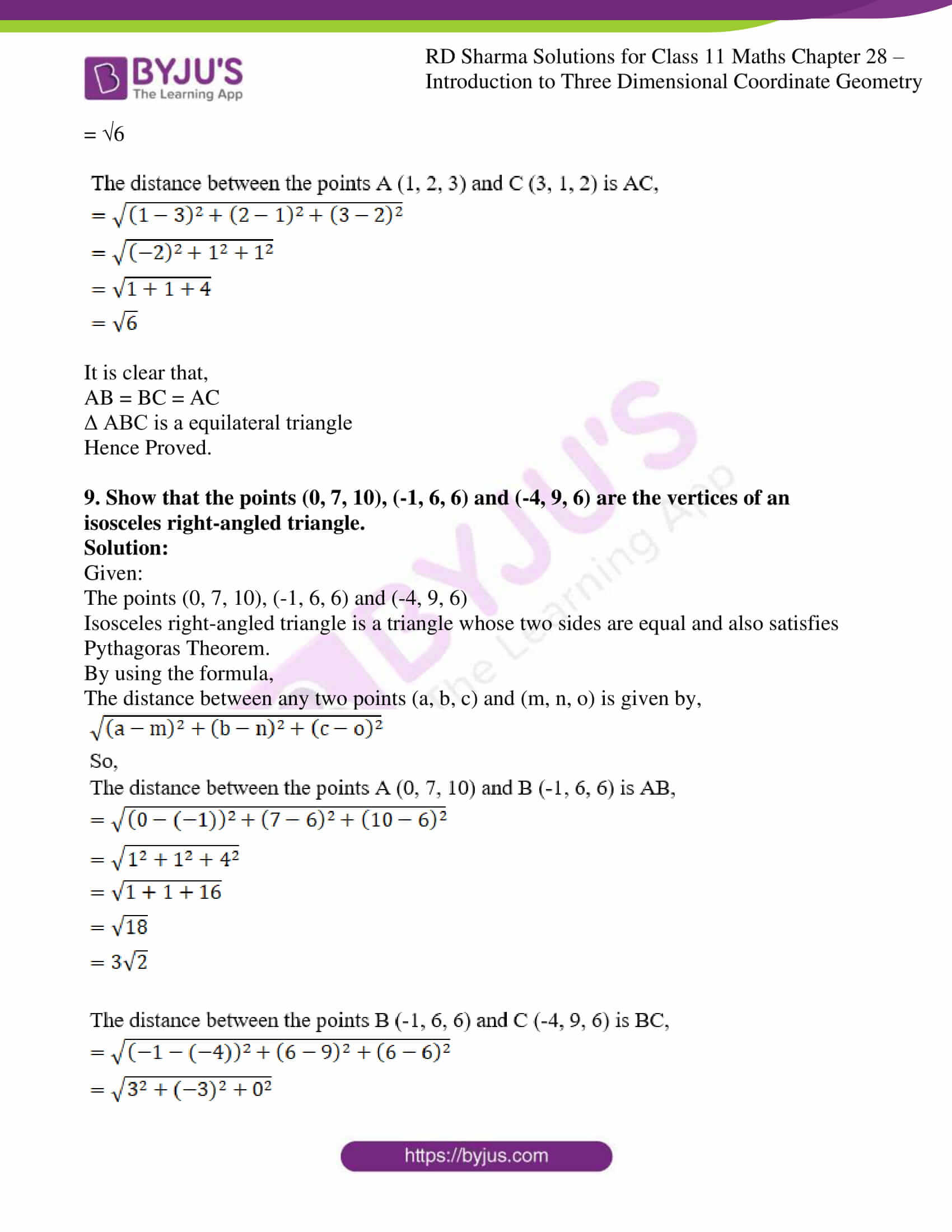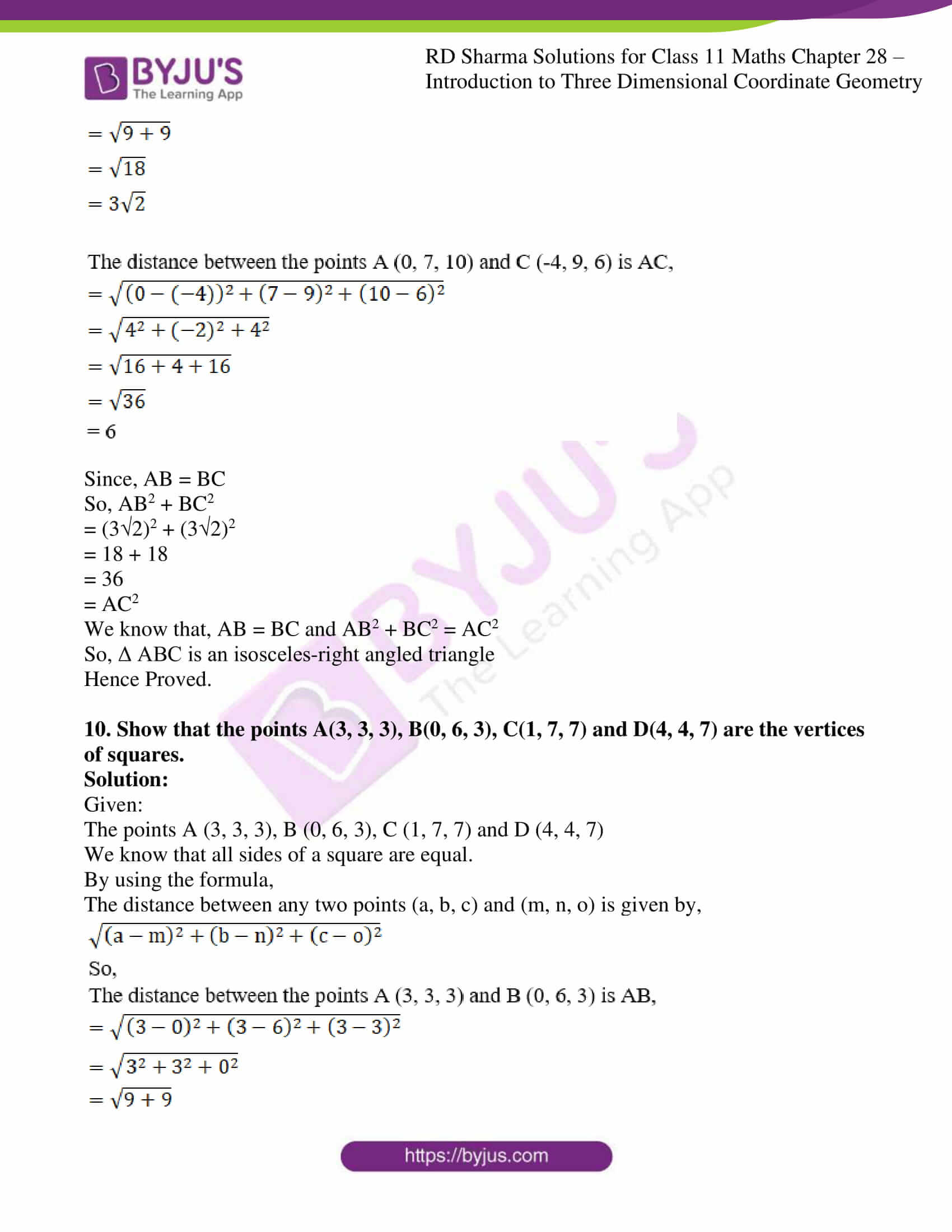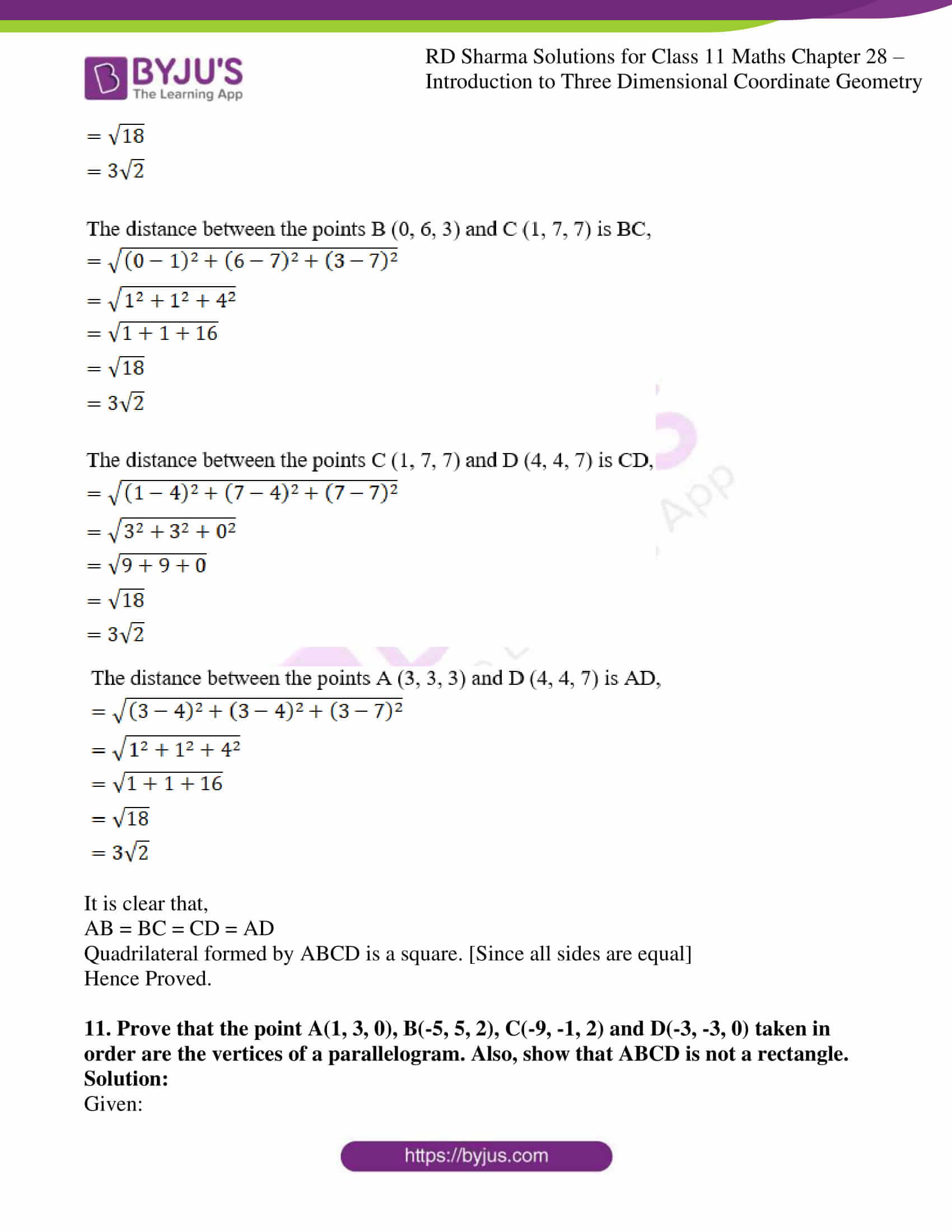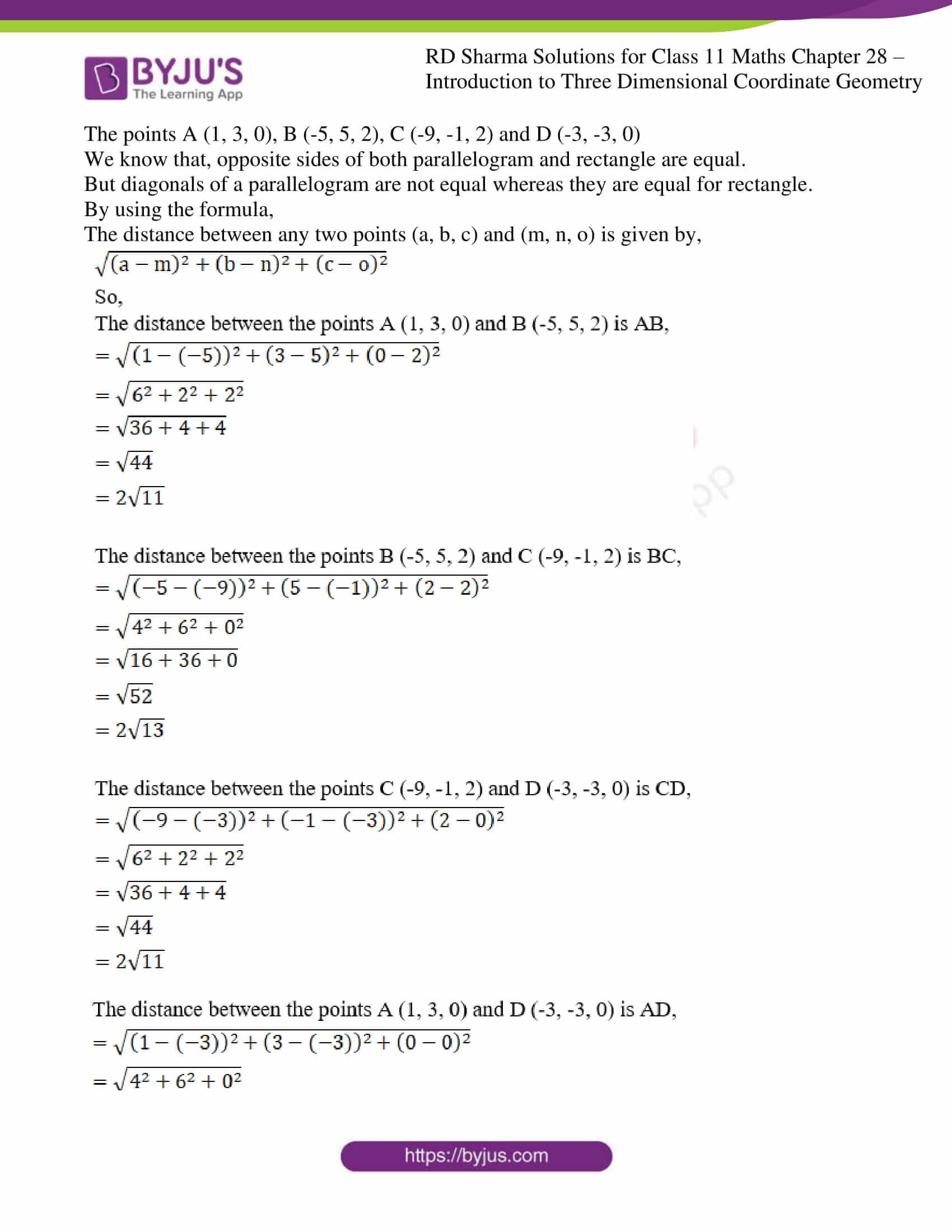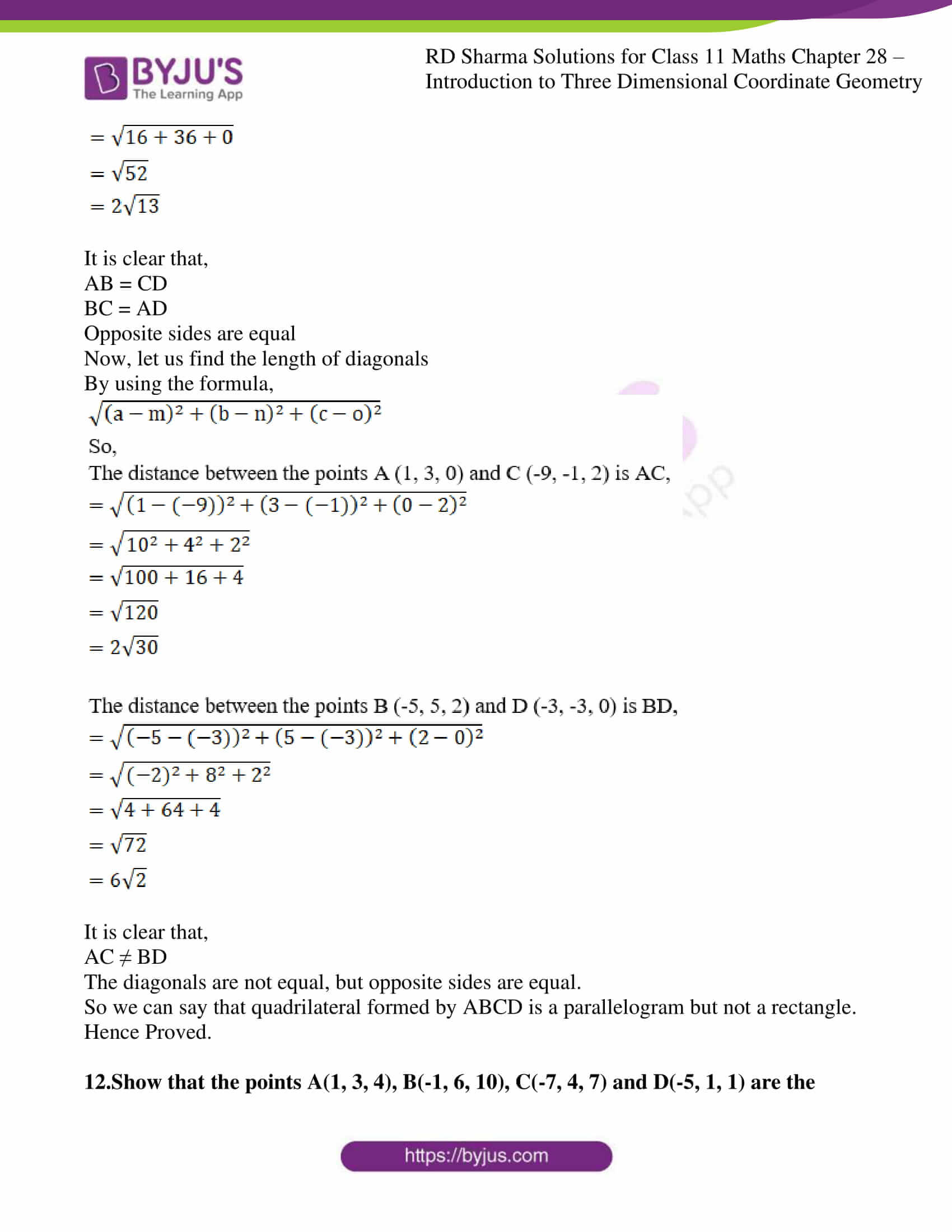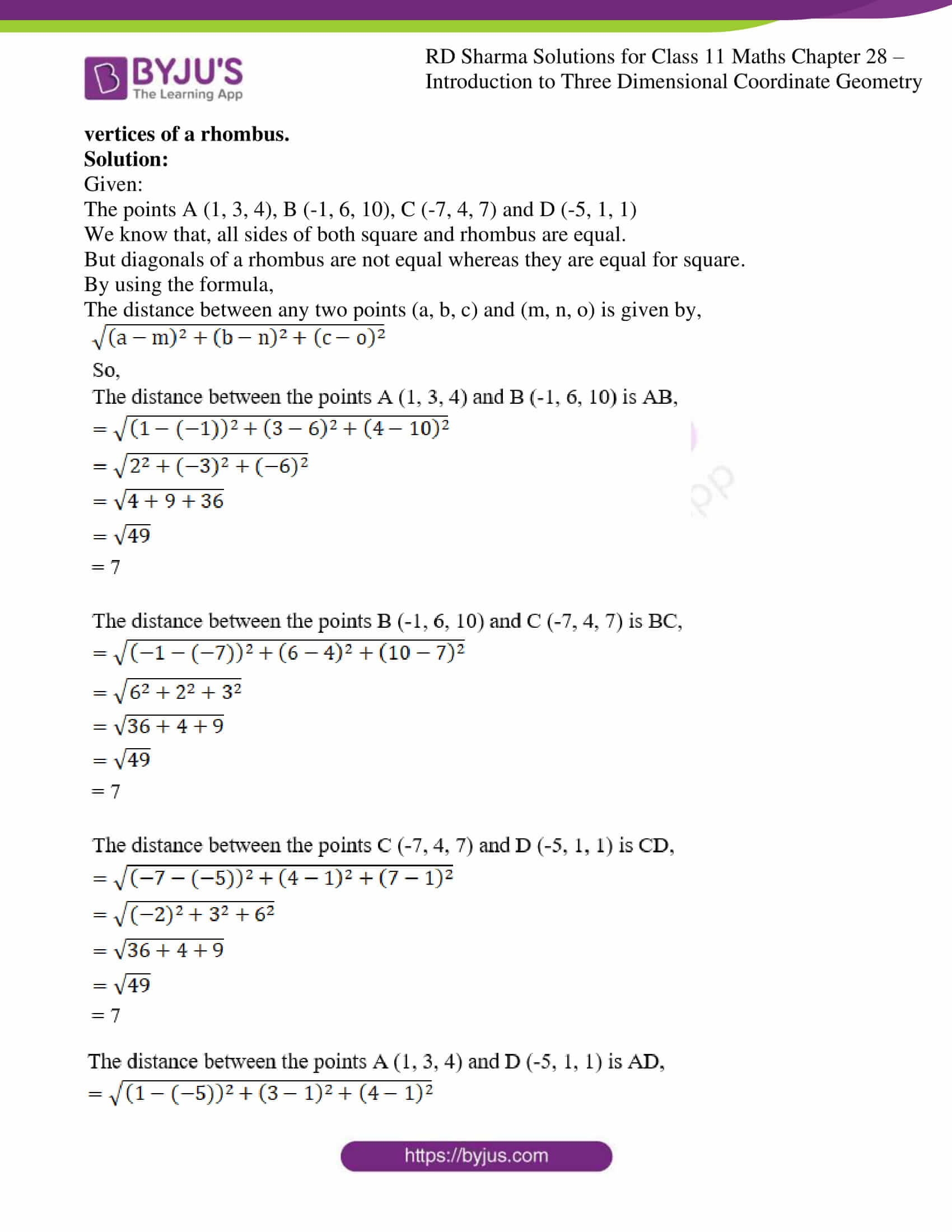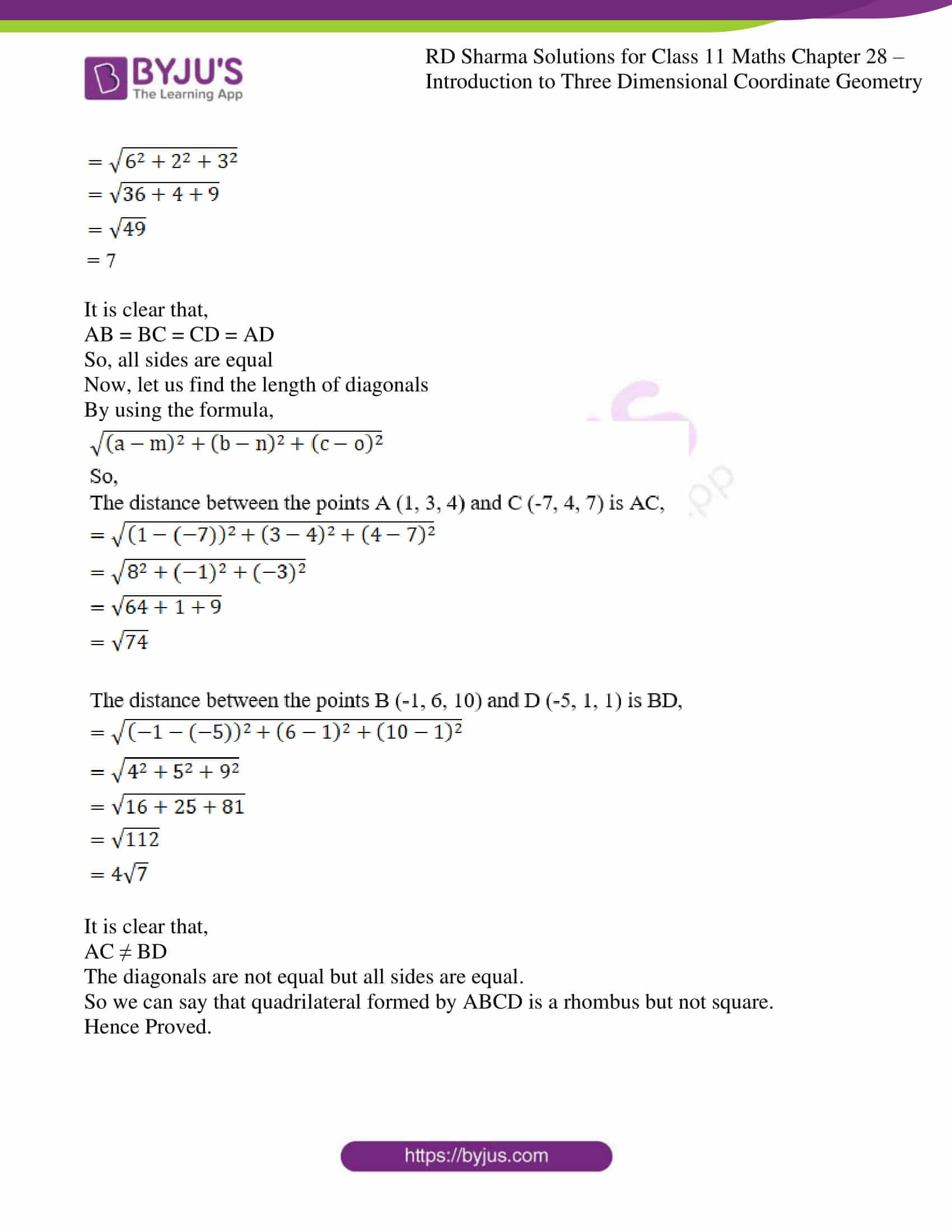### Also, access other exercises of RD Sharma Solutions for Class 11 Maths Chapter 28 – Introduction to Three Dimensional Coordinate Geometry

Exercise 28.1 Solutions

Exercise 28.3 Solutions

### Access answers to RD Sharma Solutions for Class 11 Maths Exercise 28.2 Chapter 28 – Introduction to Three Dimensional Coordinate Geometry

#### ExERCISE 28.2 PAGE NO: 28.9

1. Find the distance between the following pairs of points:
(i) P(1, -1, 0) and Q (2, 1, 2)

(ii) A(3, 2, -1) and B (-1, -1, -1)

Solution:

(i) P(1, -1, 0) and Q (2, 1, 2)

Given:

The points P(1, -1, 0) and Q (2, 1, 2)

By using the formula,

The distance between any two points (a, b, c) and (m, n, o) is given by,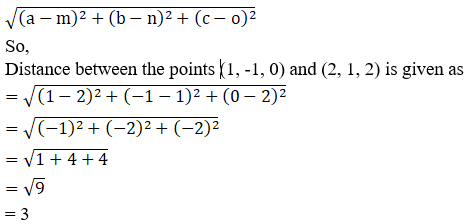∴ The Distance between P and Q is 3 units.

(ii) A (3, 2, -1) and B (-1, -1, -1)

Given:

The points A (3, 2, -1) and B (-1, -1, -1)

By using the formula,

The distance between any two points (a, b, c) and (m, n, o) is given by,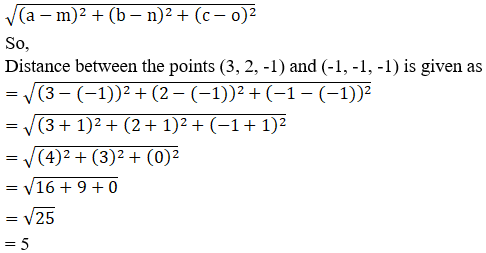= 5

∴ The Distance between A and B is 5 units.

2. Find the distance between the points P and Q having coordinates (-2, 3, 1) and (2, 1, 2).

Solution:

Given:

The points (-2, 3, 1) and (2, 1, 2)

By using the formula,

The distance between any two points (a, b, c) and (m, n, o) is given by,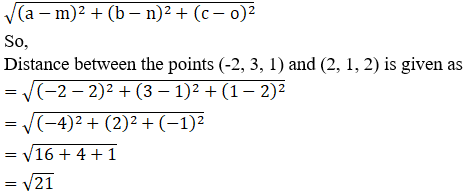∴ The Distance between the given two points is 21 units.

3. Using distance formula prove that the following points are collinear:
(i) A(4, -3, -1), B(5, -7, 6) and C(3, 1, -8)

(ii) P(0, 7, -7), Q(1, 4, -5) and R(-1, 10, -9)

(iii) A(3, -5, 1), B(-1, 0, 8) and C(7, -10, -6)
Solution:

(i) A(4, -3, -1), B(5, -7, 6) and C(3, 1, -8)

Given:

The points A(4, -3, -1), B(5, -7, 6) and C(3, 1, -8)

Points A, B and C are collinear if AB + BC = AC or AB + AC = BC or AC + BC = AB

By using the formula,

The distance between any two points (a, b, c) and (m, n, o) is given by,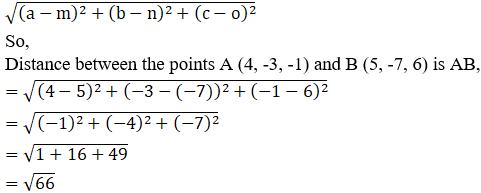= 66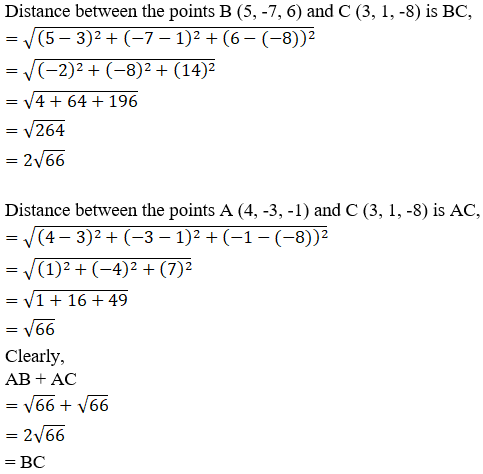∴The points A, B and C are collinear.

(ii) P (0, 7, -7), Q (1, 4, -5) and R (-1, 10, -9)

Given:

The points P (0, 7, -7), Q (1, 4, -5) and R (-1, 10, -9)

Points P, Q and R are collinear if PQ + QR = PR or PQ + PR = QR or PR + QR = PQ

By using the formula,

Distance between any two points (a, b, c) and (m, n, o) is given by,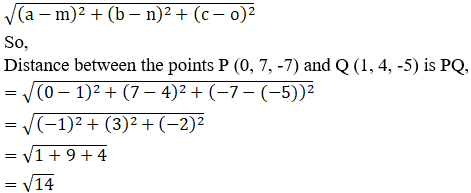= 14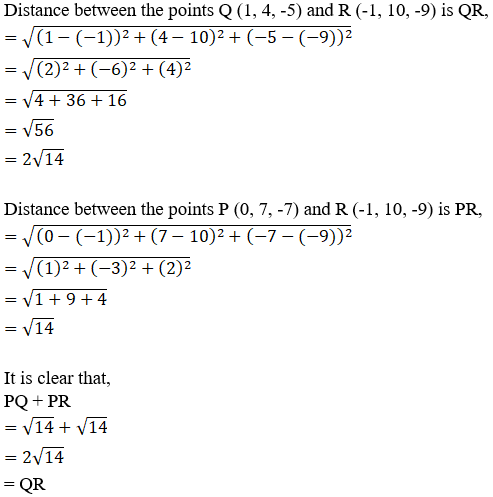∴The points P, Q and R are collinear.

(iii) A(3, -5, 1), B(-1, 0, 8) and C(7, -10, -6)

Given:

The points A(3, -5, 1), B(-1, 0, 8) and C(7, -10, -6)

Points A, B and C are collinear if AB + BC = AC or AB + AC = BC or AC + BC = AB

By using the formula,

The distance between any two points (a, b, c) and (m, n, o) is given by,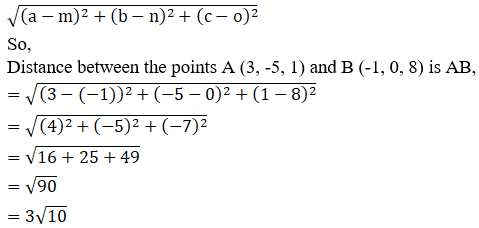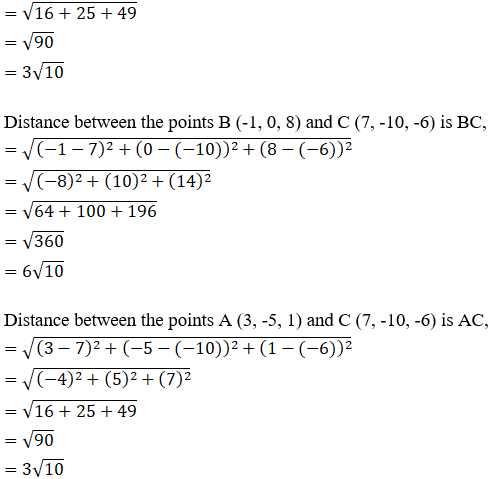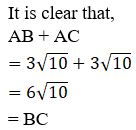∴The points A, B and C are collinear.

4. Determine the points in (i) xy-plane (ii) yz-plane and (iii) zx-plane which are equidistant from the points A(1, -1, 0), B(2, 1, 2) and C(3, 2, -1).

Solution:

Given:

The points A(1, -1, 0), B(2, 1, 2) and C(3, 2, -1)

(i) xy-plane

We know z = 0 in xy-plane.

So let P(x, y, 0) be any point in xy-plane

According to the question:

PA = PB = PC

PA2 = PB2 = PC2

By using the formula,

The distance between any two points (a, b, c) and (m, n, o) is given by,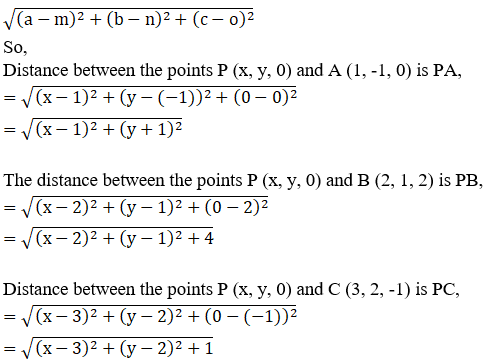We know PA2 = PB2

So, (x – 1)2+ (y + 1)2 = (x – 2)2 + (y – 1)2 + 4

x2+ 1 – 2x + y2 + 1 + 2y = x2+ 4 – 4x + y2 + 1 – 2y + 4

– 2x + 2 + 2y = 9 – 4x – 2y

– 2x + 2 + 2y – 9 + 4x + 2y = 0

2x + 4y – 7 = 0

2x = – 4y + 7……………………(1)

Since, PA2 = PC2

So, (x – 1)2+ (y + 1)2 = (x – 3)2 + (y – 2)2 + 1

x2+ 1 – 2x + y2 + 1 + 2y = x2+ 9 – 6x + y2 + 4 – 4y + 1

– 2x + 2 + 2y = 14 – 6x – 4y

– 2x + 2 + 2y – 14 + 6x + 4y = 0

4x + 6y – 12 = 0

2(2x + 3y – 6) = 0

Now substitute the value of 2x (obtained in equation (1)), we get

7 – 4y + 3y – 6 = 0

– y + 1 = 0

y = 1

By substituting the value of y back in equation (1) we get,

2x = 7 – 4y

2x = 7 – 4(1)

2x = 3

x = 3/2

∴The point P (3/2, 1, 0) in xy-plane is equidistant from A, B and C.

(ii) yz-plane

We know x = 0 in yz-plane.

Let Q(0, y, z) any point in yz-plane

According to the question:

QA = QB = QC

QA2 = QB2 = QC2

By using the formula,

The distance between any two points (a, b, c) and (m, n, o) is given by,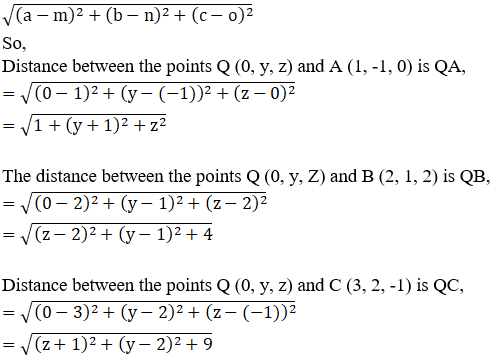We know, QA2 = QB2

So, 1 + z2+ (y + 1)2 = (z – 2)2 + (y – 1)2 + 4

z2+ 1 + y2 + 1 + 2y = z2+ 4 – 4z + y2 + 1 – 2y + 4

2 + 2y = 9 – 4z – 2y

2 + 2y – 9 + 4z + 2y = 0

4y + 4z – 7 = 0

4z = –4y + 7

z = [–4y + 7]/4 …. (1)

Since, QA2 = QC2

So, 1 + z2+ (y + 1)2 = (z + 1)2 + (y – 2)2 + 9

2+ 1 + y2 + 1 + 2y = z2+ 1 + 2z + y2 + 4 – 4y + 9

2 + 2y = 14 + 2z – 4y

2 + 2y – 14 – 2z + 4y = 0

–2z + 6y – 12 = 0

2(–z + 3y – 6) = 0

Now, substitute the value of z [obtained from (1)] we get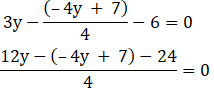12y + 4y – 7 – 24 = 0

16y – 31 = 0

y = 31/16

Substitute the value of y back in equation (1), we get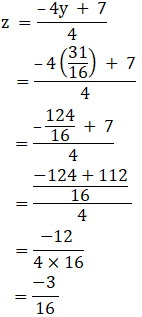= -3/16

∴The point Q (0, 31/16, -3/16) in yz-plane is equidistant from A, B and C.

(iii) zx-plane

We know y = 0 in xz-plane.

Let R(x, 0, z) any point in xz-plane

According to the question:

RA = RB = RC

RA2 = RB2 = RC2

By using the formula,

The distance between any two points (a, b, c) and (m, n, o) is given by,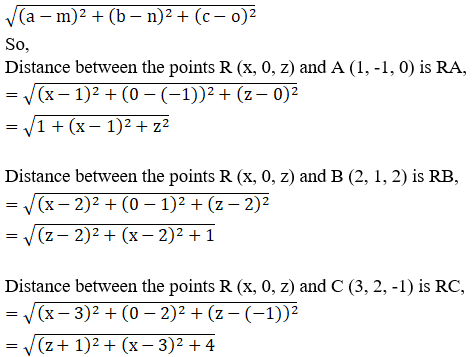We know, RA2 = RB2

So, 1 + z2+ (x – 1)2 = (z – 2)2 + (x – 2)2 + 1

z2+ 1 + x2 + 1 – 2x = z2+ 4 – 4z + x2 + 4 – 4x + 1

2 – 2x = 9 – 4z – 4x

2 + 4z – 9 + 4x – 2x = 0

2x + 4z – 7 = 0

2x = –4z + 7……………………………(1)

Since, RA2 = RC2

So, 1 + z2+ (x – 1)2 = (z + 1)2 + (x – 3)2 + 4

z2+ 1 + x2 + 1 – 2x = z2+ 1 + 2z + x2 + 9 – 6x + 4

2 – 2x = 14 + 2z – 6x

2 – 2x – 14 – 2z + 6x = 0

–2z + 4x – 12 = 0

2(2x) = 12 + 2z

Substitute the value of 2x [obtained from equation (1)] we get,

2(–4z + 7) = 12 + 2z

–8z + 14 = 12 + 2z

14 – 12 = 8z + 2z

10z = 2

z = 2/10

= 1/5

Now, substitute the value of z back in equation (1), we get

2x = -4z + 7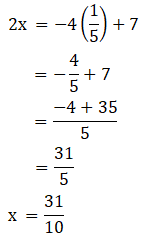∴The point R (31/10, 0, 1/5) in xz-plane is equidistant from A, B and C.

5. Determine the point on z-axis which is equidistant from the points (1, 5, 7) and (5, 1, -4)

Solution:

Given:

The points (1, 5, 7) and (5, 1, -4)

We know x = 0 and y = 0 on z-axis

Let R(0, 0, z) any point on z-axis

According to the question:

RA = RB

RA2 = RB2

By using the formula,

The distance between any two points (a, b, c) and (m, n, o) is given by,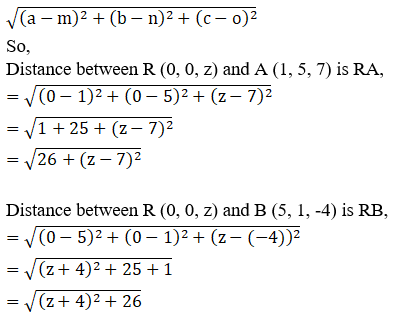We know, RA2 = RB2

26+ (z – 7)2 = (z + 4)2 + 26

z2+ 49 – 14z + 26 = z2+ 16 + 8z + 26

49 – 14z = 16 + 8z

49 – 16 = 14z + 8z

22z = 33

z = 33/22

= 3/2

∴The point R (0, 0, 3/2) on z-axis is equidistant from (1, 5, 7) and (5, 1, -4).

6. Find the point on y-axis which is equidistant from the points (3, 1, 2) and (5, 5, 2).

Solution:

Given:

The points (3, 1, 2) and (5, 5, 2)

We know x = 0 and z = 0 on y-axis

Let R(0, y, 0) any point on the y-axis

According to the question:

RA = RB

RA2 = RB2

By using the formula,

The distance between any two points (a, b, c) and (m, n, o) is given by,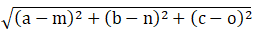So,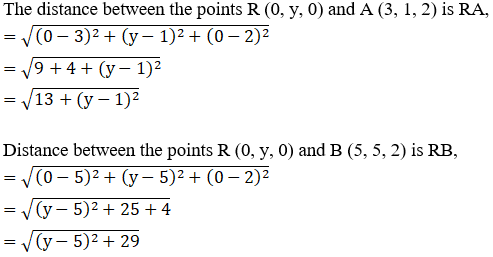We know, RA2 = RB2

13+ (y – 1)2 = (y – 5)2 + 29

y2+ 1 – 2y + 13 = y2+ 25 – 10y + 29

10y – 2y = 54 – 14

8y = 40

y = 40/8

= 5

∴The point R (0, 5, 0) on y-axis is equidistant from (3, 1, 2) and (5, 5, 2).

7. Find the points on z-axis which are at a distance√21 from the point (1, 2, 3).

Solution:

Given:

The point (1, 2, 3)

Distance = √21

We know x = 0 and y = 0 on z-axis

Let R(0, 0, z) any point on z-axis

According to question:

RA = √21

RA2 = 21

By using the formula,

The distance between any two points (a, b, c) and (m, n, o) is given by,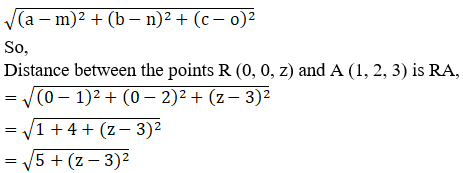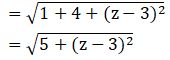We know, RA2 = 21

5 + (z – 3)2 = 21

z2+ 9 – 6z + 5 = 21

z2 – 6z = 21 – 14

z2– 6z – 7 = 0

z2– 7z + z – 7 = 0

z(z– 7) + 1(z – 7) = 0

(z– 7) (z + 1) = 0

(z– 7) = 0 or (z + 1) = 0

z= 7 or z = -1

∴The points (0, 0, 7) and (0, 0, -1) on z-axis is equidistant from (1, 2, 3).

8. Prove that the triangle formed by joining the three points whose coordinates are (1, 2, 3), (2, 3, 1) and (3, 1, 2) is an equilateral triangle.

Solution:

Given:

The points (1, 2, 3), (2, 3, 1) and (3, 1, 2)

An equilateral triangle is a triangle whose all sides are equal.

So let us prove AB = BC = AC

By using the formula,

The distance between any two points (a, b, c) and (m, n, o) is given by,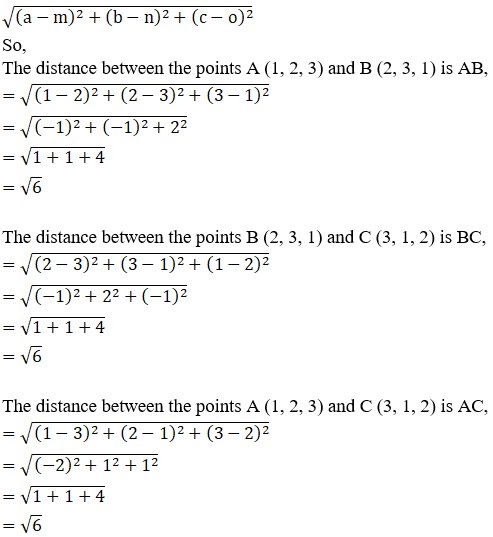= 6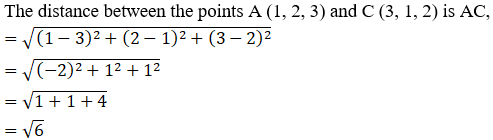It is clear that,

AB = BC = AC

Δ ABC is a equilateral triangle

Hence Proved.

9. Show that the points (0, 7, 10), (-1, 6, 6) and (-4, 9, 6) are the vertices of an isosceles right-angled triangle.

Solution:

Given:

The points (0, 7, 10), (-1, 6, 6) and (-4, 9, 6)

Isosceles right-angled triangle is a triangle whose two sides are equal and also satisfies Pythagoras Theorem.

By using the formula,

The distance between any two points (a, b, c) and (m, n, o) is given by,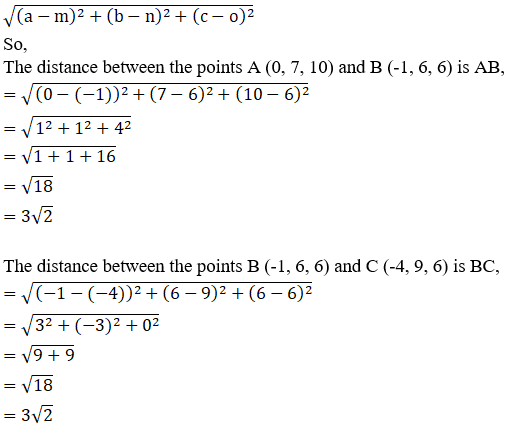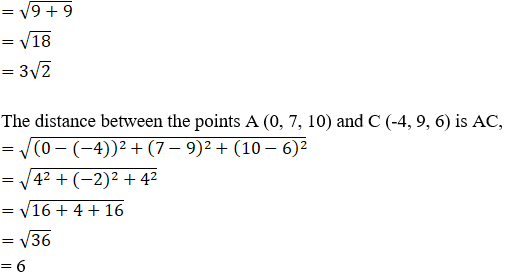Since, AB = BC

So, AB2 + BC2

= (32)2 + (32)2

= 18 + 18

= 36

= AC2

We know that, AB = BC and AB2 + BC2 = AC2

So, Δ ABC is an isosceles-right angled triangle

Hence Proved.

10. Show that the points A(3, 3, 3), B(0, 6, 3), C(1, 7, 7) and D(4, 4, 7) are the vertices of squares.

Solution:

Given:

The points A (3, 3, 3), B (0, 6, 3), C (1, 7, 7) and D (4, 4, 7)

We know that all sides of a square are equal.

By using the formula,

The distance between any two points (a, b, c) and (m, n, o) is given by,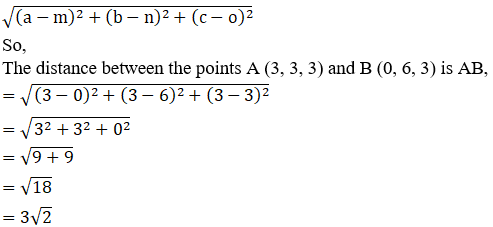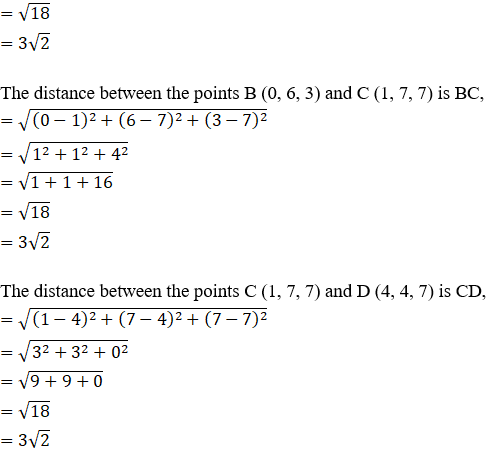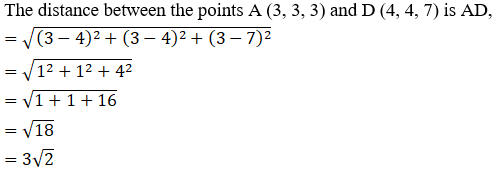It is clear that,

AB = BC = CD = AD

Quadrilateral formed by ABCD is a square. [Since all sides are equal]

Hence Proved.

11. Prove that the point A(1, 3, 0), B(-5, 5, 2), C(-9, -1, 2) and D(-3, -3, 0) taken in order are the vertices of a parallelogram. Also, show that ABCD is not a rectangle.

Solution:

Given:

The points A (1, 3, 0), B (-5, 5, 2), C (-9, -1, 2) and D (-3, -3, 0)

We know that, opposite sides of both parallelogram and rectangle are equal.

But diagonals of a parallelogram are not equal whereas they are equal for rectangle.

By using the formula,

The distance between any two points (a, b, c) and (m, n, o) is given by,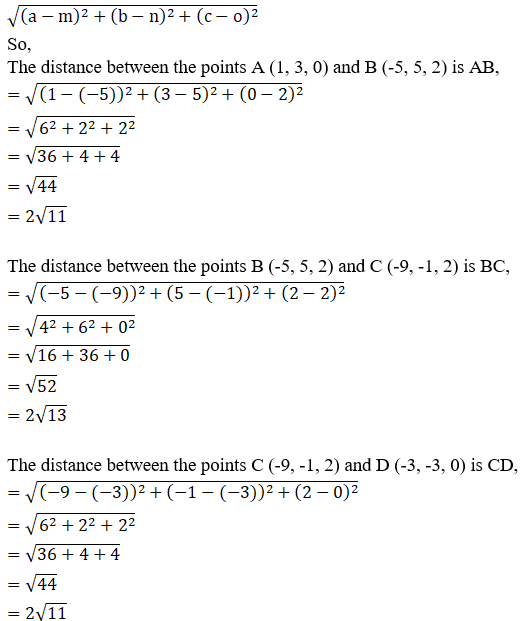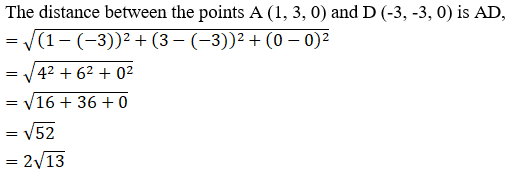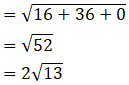It is clear that,

AB = CD

Opposite sides are equal

Now, let us find the length of diagonals

By using the formula,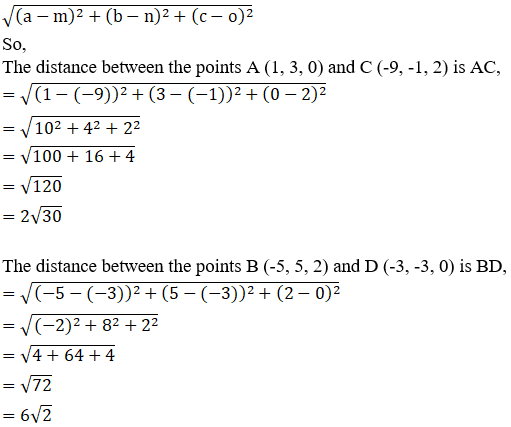It is clear that,

AC ≠ BD

The diagonals are not equal, but opposite sides are equal.

So we can say that quadrilateral formed by ABCD is a parallelogram but not a rectangle.

Hence Proved.

12.Show that the points A(1, 3, 4), B(-1, 6, 10), C(-7, 4, 7) and D(-5, 1, 1) are the vertices of a rhombus.

Solution:

Given:

The points A (1, 3, 4), B (-1, 6, 10), C (-7, 4, 7) and D (-5, 1, 1)

We know that, all sides of both square and rhombus are equal.

But diagonals of a rhombus are not equal whereas they are equal for square.

By using the formula,

The distance between any two points (a, b, c) and (m, n, o) is given by,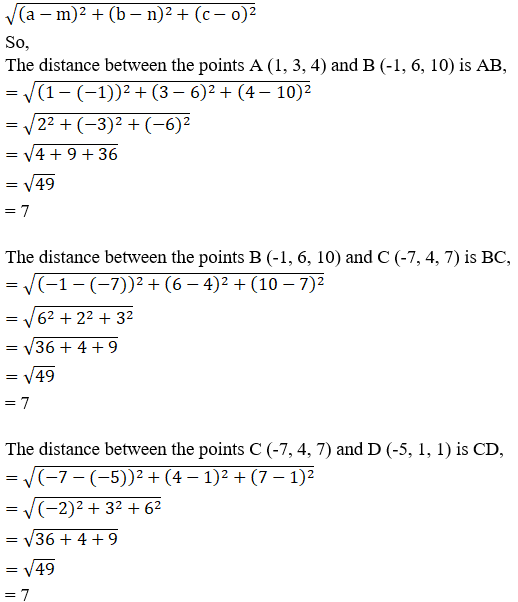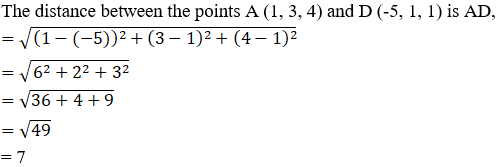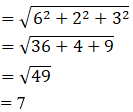It is clear that,

AB = BC = CD = AD

So, all sides are equal

Now, let us find the length of diagonals

By using the formula,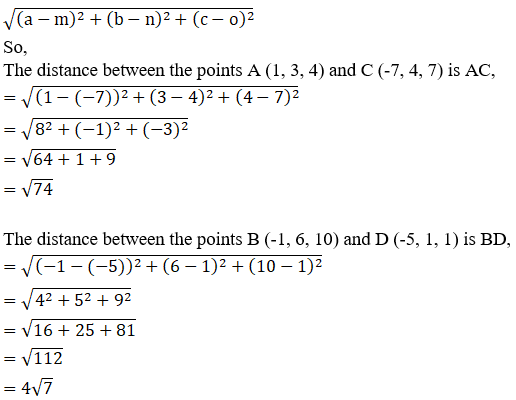It is clear that,

AC ≠ BD

The diagonals are not equal but all sides are equal.

So we can say that quadrilateral formed by ABCD is a rhombus but not square.

Hence Proved.